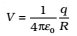# Electrostatic Potential – Definition, SI Unit and Formula

0
175

## What is Electrostatic Potential?

The amount of work done to move a unit charge from a reference point to a specific point inside the field without producing an acceleration.

## Electric Potential Formula= electric potential= Coulomb constant= charge= distance of separation

### SI Unit of Electrostatic Potential

The SI unit of electrostatic potential is volt.

 SI unit of electrostatic potential volt Other units statvolt Symbol of electrostatic potential V or φ Dimensional formula ML2T3I-1

We know that central forces are conservative in nature i.e., work done on any particle moving under the influence of conservative forces does not depend on path taken by the particle but depends on initial and final positions of the particle.

Electrostatic force given by Coulomb’s law is also a central force like gravitational force and is conservative in nature.

For conservative forces, work done on particle undergoing displacement can be expressed in terms of the potential energy function.

## Electrostatic Potential

Work done per unit test charge is characteristic of the electric field associated with the charge configuration. This leads to the idea of electrostatic potential V due to a given charge configuration.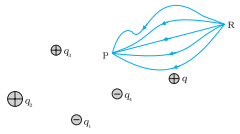Work done by external force in bringing a unit positive charge from point R to P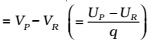where VP and VR are the electrostatic potentials at P and R, respectively.

Work done by an external force in bringing a unit positive charge from infinity to a point = electrostatic potential (V) at that point.

In other words, the electrostatic potential (V) at any point in a region with electrostatic field is the work done in bringing a unit positive charge (without acceleration) from infinity to that point.

SI unit of Potential difference is Volt. 1V=1Nm C-1.

### Potential Due to a Point Charge

Consider a point charge Q at the origin. For Q > 0, the work done against the repulsive force on the test charge is positive. Choose a convenient path along the radial direction from infinity to the point P.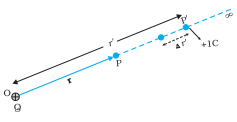At some intermediate point P′ on the path, the electrostatic force on a unit positive charge is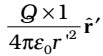where rˆ′ is the unit vector along OP′. Work done against this force from r′ to r′ + ∆r′ is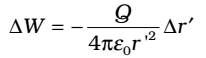The negative sign appears because for ∆r′ < 0, ∆W is positive. Total work done (W) by the external force is obtained by integrating from r′ = ∞ to r′ = r,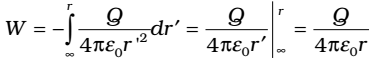This, by definition, is the potential at P due to the charge Q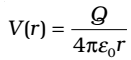Figure below shows how the electrostatic potential ( ∝ 1/r) and the electrostatic field ( ∝ 1/r2) varies with r.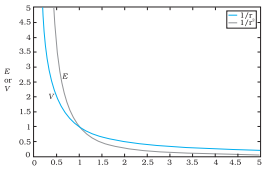### Potential Due to an Electric Dipole

Take the origin at the center of the dipole. Since the potential is related to the work done by the field, the electrostatic potential also follows the superposition principle. Thus, the potential due to the dipole is the sum of potentials due to the charges q and –q.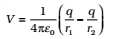where r1 and r2 are the distance of the point from q and –q, respectively.

Now, by geometry,Take r much greater than a (r ≫ a) and retain terms only up to the first order in a/r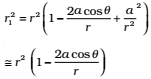Similarly,Using the Binomial theorem and retaining terms up to the first order in a/r; obtain,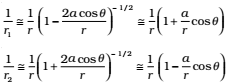Using Equations and p = 2qa, we get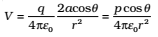Now,

where is the unit vector along the position vector OP. The electric potential of a dipole is then given by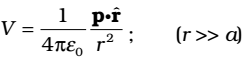The equation is approximately true only for distances large compared to the size of the dipole so that higher order terms in a/r are negligible. For a point dipole p at the origin,

From Eq. the potential on the dipole axis (θ= 0, π) is given by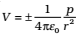(Positive sign for θ= 0, negative sign for θ= π.) The potential in the equatorial plane (θ= π/2) is zero.

1. The potential due to a dipole depends not just on r but also on the angle between the position vector r and the dipole moment vector p.
2. The electric dipole potential falls off, at large distance, as 1/r2, not as 1/r, characteristic of the potential due to a single charge

### Potential due to a system of charges

Consider a system of charges q1, q2,…, qn with position vectors r1, r2,…, rn relative to some origin. The potential V1 at P due to the charge q1 is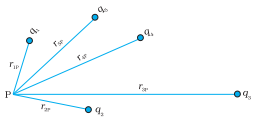where r1P is the distance between q and P. Similarly, the potential V2 at P due to q2 and due to q are given by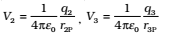where r2P and r3P are the distances of P from charges qand q3, respectively; and so on for the potential due to other charges.

By the superposition principle, the potential V at P due to the total charge configuration is the algebraic sum of the potentials due to the individual charges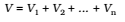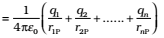The electric field outside the shell is as if the entire charge is concentrated at the centre. Thus, the potential outside the shell is given by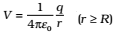where q is the total charge on the shell and R its radius. The electric field inside the shell is zero. This implies that potential is constant inside the shell (as no work is done in moving a charge inside the shell), and, therefore, equals its value at the surface, which is